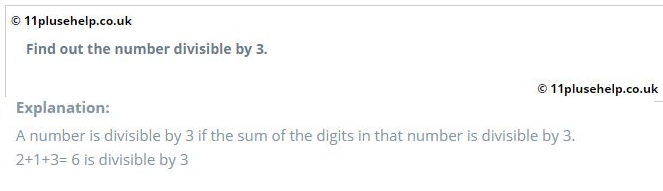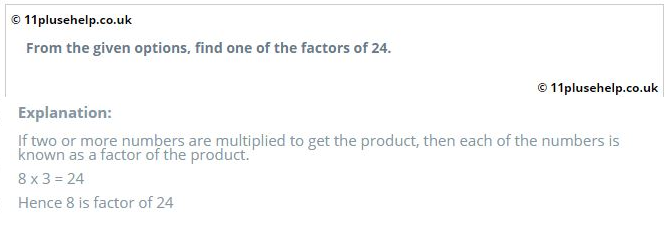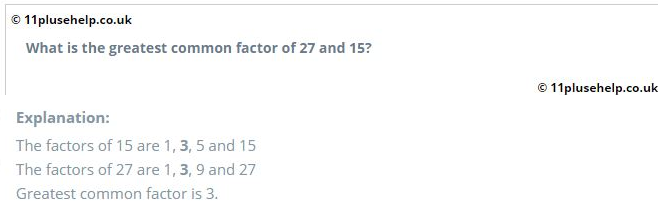Latest Results:

# 11 Plus Factors

While calculating the Least common factor(LCM) and the Highest common factor, we need to list out all the factors of the numbers given. So it is compulsory to learn how to find the factors of the given numbers.

A number is said to be a factor of the second number if the first number divides the second number without leaving the remainder. In the case of the division with zero remainders, the divisor is a factor of dividend.

For example,

12 ÷ 4 = 3, remainder = 0

So, 4 is the factor of 12.

Properties related to factors:

1. ‘1’ is the factor of all positive numbers.
2. Every Number is a factor of itself.
3. Every factor should less than or equal to the Number.
4. The number of factors of any number is finite
5. Prime Number has only two factors.

Key points to remember:

• If the last digit of any number is 0 or 2 or 4 or 6 or 8, then the number is divisible by 2.
• A number is divisible by three if the sum of the digits in that number is divisible by 3.
• If the last two digits of any number are multiples of 4, then the number is divisible by 4
• If the last digit of any number is 0 or 5, then the number is divisible by 5.
• If any number is divisible by both 2 and 3, then the number is divisible by 6
• If the last three digits of any number are multiples of 8, then the number is divisible by 8
• A number is divisible by nine if the sum of the digits in that number is divisible by 9.
• If the last digit of any number is 0, then the number is divisible by 10.
• The Sum of even digits is subtracted from the sum of odd digits. If the result is ‘0’ or ‘11’, the number is divisible by 11.
• If any number is divisible by both 3 and 4, then the number is divisible by 12.

In 11 plus maths papers, maths topics like LCM and HCF are related to factors. So the practice of questions on the 11 plus factors topic is necessary.

Let us have some examples:

Example:1Example:2Example:3You can access 11 plus practice papers on different topics here:

https://www.11plusehelp.co.uk/11-plus-maths-practice-tests

Free11 plus practice papers link :

11PluseHelp.co.uk also provides you with free 11 plus maths papers. 11 plus maths papers free download available in below link:

11PluseHelp.co.uk is a one-stop shop for 11 Plus exam preparation, including 11 Plus Independent schools preparation.

There are many 11 Plus practice papers for entrance exams, Independent school papers and more for the 11 test with us. Ranging from 10 to 25 minutes, our multiple-choice and fill-up question types are ideal for Maths revision.

At 11plusehelp.co.uk, we possess the following resources:

Maths- 11 Plus Maths Worksheets, 11 Plus Maths Papers free download, 11 Plus Maths topics pdfs.

English- 11 Plus English papers with answers, 11 Plus comprehension with answers, 11 English comprehensions multiple-choice, English reasoning test.

Verbal and Non-Verbal Reasoning- 11 Plus Verbal Reasoning worksheets free, Verbal and Non-Verbal Reasoning tests, easy Non-Verbal Reasoning Worksheets

Practice Tests- Eleven plus sample papers, free 11 plus papers with answers, 11 plus mock test papers pdf, 11 exam question and answers, UK 11 plus exam questions, 11 online tests in Maths and English with 11 Plus answers.

We also have 11 plus resources for parents with 11 plus guide.

Our Independent School resources include-

Grammar school test papers with answers

KENT- KENT test practice papers, KENT 11 Plus

Bexley - Bexley 11 Plus Test, Bexley 11 Plus Solved Papers, Bexley 11 Plus Comprehension with Answers

KS2- Maths Reasoning KS2, Online Math Test KS2

CEM- CEM assessments, CEM 11 papers pdf, CEM 11 Familiarization papers:https://www.11plusehelp.co.uk/11-plus-exam-papers/11-plus-cem-exam

GL assessment - https://www.11plusehelp.co.uk/11-plus-gl-assessment-exam

11 Plus Mock Tests Berkshire, 11 Plus Mock Exams Essex, Buckinghamshire 11 Plus 2019 Solved

Top 100 Independent School Details:https://www.11plusehelp.co.uk/top-100-independent-schools

With all this preparation, Revision pack, and guide free advice, pack your bags to the grammar school/ private school you dream of.

11 Plus Revision Pack:

https://www.11plusehelp.co.uk/11-plus-practice-papers/11-plus-english-practice-test-papers/revision-pack

We focus on fundamentals, logic, basics and cover most of the syllabus for all 11 Plus exam patterns in the UK. For example, We cover Maths topic wise questions and fundamentals and how to apply them. We have constantly been adding practice papers regularly.

We have loads of questions useful for the preparation of CEM, CSSE and GL assessment and any other 11 Plus entrance exams in the UK. Children will love to take these tests, and learning is fun at 11plusehelp.co.uk.

To access Virtual Mock Exams, please visit:  https://11plus-mockexams.co.uk/

You can access 11 Plus FREE Papers by visiting the below link:
https://www.11plusehelp.co.uk/11-plus-free-online-papers

You can access 11 Plus FREE Sample Papers by visiting the below link:
https://www.11plusehelp.co.uk/11-plus-sample-papers

11 Plus complete solution features:

https://11plusehelp.co.uk/blog/2017/05/01/11-plus-complete-solution/

Practice and Perseverance Over Genius and Talent

Thanks,

11Plusehelp.co.uk